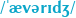# on 09-Jan-2020 (Thu)

#### Annotation 4763882229004

#distance #straight-lines

Because the lines are parallel, the perpendicular distance between them is a constant, so it does not matter which point is chosen to measure the distance. Given the equations of two non-vertical parallel lines

$$y=mx+b_{1}\,$$ $$y=mx+b_{2}\,,$$

the distance between the two lines is the distance between the two intersection points of these lines with the perpendicular line

$$y=-x/m\,.$$

This distance can be found by first solving the linear systems

$${\begin{cases}y=mx+b_{1}\\y=-x/m\,,\end{cases}}$$

and

$${\begin{cases}y=mx+b_{2}\\y=-x/m\,,\end{cases}}$$

to get the coordinates of the intersection points. The solutions to the linear systems are the points

$$\left(x_{1},y_{1}\right)\ =\left({\frac {-b_{1}m}{m^{2}+1}},{\frac {b_{1}}{m^{2}+1}}\right)\,,$$

and

$$\left(x_{2},y_{2}\right)\ =\left({\frac {-b_{2}m}{m^{2}+1}},{\frac {b_{2}}{m^{2}+1}}\right)\,.$$

The distance between the points is

$$d={\sqrt {\left({\frac {b_{1}m-b_{2}m}{m^{2}+1}}\right)^{2}+\left({\frac {b_{2}-b_{1}}{m^{2}+1}}\right)^{2}}}\,,$$

which reduces to

$$d={\frac {|b_{2}-b_{1}|}{{\sqrt {m^{2}+1}}}}\,.$$

When the lines are given by

$$ax+by+c_{1}=0\,$$ $$ax+by+c_{2}=0,\,$$

the distance between them can be expressed as

$$d={\frac {|c_{2}-c_{1}|}{{\sqrt {a^{2}+b^{2}}}}}.$$

Distance between two straight lines - Wikipedia
el lines, the distance is the perpendicular distance from any point on one line to the other line. Contents 1 Formula and proof 2 See also 3 References 4 External links Formula and proof[edit ] <span>Because the lines are parallel, the perpendicular distance between them is a constant, so it does not matter which point is chosen to measure the distance. Given the equations of two non-vertical parallel lines y = m x + b 1 {\displaystyle y=mx+b_{1}\,} y = m x + b 2 , {\displaystyle y=mx+b_{2}\,,} the distance between the two lines is the distance between the two intersection points of these lines with the perpendicular line y = − x / m . {\displaystyle y=-x/m\,.} This distance can be found by first solving the linear systems { y = m x + b 1 y = − x / m , {\displaystyle {\begin{cases}y=mx+b_{1}\\y=-x/m\,,\end{cases}}} and { y = m x + b 2 y = − x / m , {\displaystyle {\begin{cases}y=mx+b_{2}\\y=-x/m\,,\end{cases}}} to get the coordinates of the intersection points. The solutions to the linear systems are the points ( x 1 , y 1 ) = ( − b 1 m m 2 + 1 , b 1 m 2 + 1 ) , {\displaystyle \left(x_{1},y_{1}\right)\ =\left({\frac {-b_{1}m}{m^{2}+1}},{\frac {b_{1}}{m^{2}+1}}\right)\,,} and ( x 2 , y 2 ) = ( − b 2 m m 2 + 1 , b 2 m 2 + 1 ) . {\displaystyle \left(x_{2},y_{2}\right)\ =\left({\frac {-b_{2}m}{m^{2}+1}},{\frac {b_{2}}{m^{2}+1}}\right)\,.} The distance between the points is d = ( b 1 m − b 2 m m 2 + 1 ) 2 + ( b 2 − b 1 m 2 + 1 ) 2 , {\displaystyle d={\sqrt {\left({\frac {b_{1}m-b_{2}m}{m^{2}+1}}\right)^{2}+\left({\frac {b_{2}-b_{1}}{m^{2}+1}}\right)^{2}}}\,,} which reduces to d = | b 2 − b 1 | m 2 + 1 . {\displaystyle d={\frac {|b_{2}-b_{1}|}{\sqrt {m^{2}+1}}}\,.} When the lines are given by a x + b y + c 1 = 0 {\displaystyle ax+by+c_{1}=0\,} a x + b y + c 2 = 0 , {\displaystyle ax+by+c_{2}=0,\,} the distance between them can be expressed as d = | c 2 − c 1 | a 2 + b 2 . {\displaystyle d={\frac {|c_{2}-c_{1}|}{\sqrt {a^{2}+b^{2}}}}.} See also[edit ] Distance from a point to a line Skew lines#Distance References[edit ] Abstand In: Schülerduden – Mathematik II. Bibliographisches Institut & F. A. Brockhaus, 2004, I

#### Annotation 4768374590732

#Médecine #Pathophysiology-Of-Disease #Physiologie
Most preparations of estrogen and progestin block the LH surge at midcycle, thereby preventing ovulation. However, other contraceptive actions include effects on estrogen- and progesterone-sensitive tissues, such as inducing antifertility changes in cervical mucus and the endometrial lining that are unfavorable to sperm transport and embryonic implantation, respectively.

#### pdf

cannot see any pdfs

#### Annotation 4769605356812

#MLBook #building-blocks #fundamental-algorithms #machine-learning
In this chapter, I describe five algorithms which are not just the most known but also either very effective on their own or are used as building blocks for the most effective learning algorithms out there.

#### pdf

cannot see any pdfs

#### Annotation 4769608502540

#MLBook #linear-regression #machine-learning
Linear regression is a popular regression learning algorithm that learns a model which is a linear combination of features of the input example.

#### pdf

cannot see any pdfs

#### Annotation 4769615056140

#nn
Artificial neural networks (ANN) or connectionist systems

Artificial neural network - Wikipedia
lification of neurons in a brain. Here, each circular node represents an artificial neuron and an arrow represents a connection from the output of one artificial neuron to the input of another. <span>Artificial neural networks (ANN) or connectionist systems are computing systems vaguely inspired by the biological neural networks that constitute animal brains. Such systems "learn" to perform tasks by considering examples, generally witho

#### Annotation 4769617677580

#MLBook #linear-regression #machine-learning #problem-statement

We have a collection of labeled examples $$\{ ( \mathbf x_i , y_i ) \}^N_{i=1}$$ , where $$N$$ is the size of the collection, $$\mathbf x_i$$ is the $$D$$-dimensional feature vector of example $$i = 1 , . . . , N$$ , $$y_i$$ is a real-valued target and every feature $$x^{(j)}_i , j = 1, \ldots , D$$, is also a real number. We want to build a model $$f_{\mathbf w,b} (\mathbf x)$$ as a linear combination of features of example $$\mathbf x$$:

$$f_{\mathbf w,b} (\mathbf x) = \mathbf w \mathbf x + b$$,

where $$\mathbf w$$ is a $$D$$-dimensional vector of parameters and $$b$$ is a real number. The notation $$f_{\mathbf w,b} (\mathbf x)$$ means that the model $$f$$ is parametrized by two values: $$\mathbf w$$ and $$\mathbf b$$.

We will use the model to predict the unknown $$y$$ for a given $$\mathbf x$$ like this: $$y \leftarrow f_{\mathbf w,b} ( x )$$. Two models parametrized by two different pairs $$( \mathbf w, b )$$ will likely produce two different predictions when applied to the same example. We want to find the optimal values $$( \mathbf w^\ast, b^\ast )$$. Obviously, the optimal values of parameters define the model that makes the most accurate predictions.

#### pdf

cannot see any pdfs

#### Annotation 4769620036876

[unknown IMAGE 4769622658316]
#MLBook #has-images #linear-regression #machine-learning

You could have noticed that the form of our linear model in eq. 1 $$\left[ f_{\mathbf w,b} (\mathbf x) = \mathbf w \mathbf x + b \right]$$ is very similar to the form of the SVM model. The only difference is the missing sign operator. The two models are indeed similar. However, the hyperplane in the SVM plays the role of the decision boundary: it’s used to separate two groups of examples from one another. As such, it has to be as far from each group as possible.

On the other hand, the hyperplane in linear regression is chosen to be as close to all training examples as possible.

You can see why this latter requirement is essential by looking at the illustration in Figure 1. It displays the regression line (in red) for one-dimensional examples (blue dots). We can use this line to predict the value of the target $$y$$ new for a new unlabeled input example $$x_{new}$$ new . If our examples are $$D$$-dimensional feature vectors (for $$D > 1$$), the only difference with the one-dimensional case is that the regression model is not a line but a plane (for two dimensions) or a hyperplane (for $$D > 2$$).

#### pdf

cannot see any pdfs

#### Annotation 4769626066188

#MLBook #cost-function #empirical-risk #linear-regression #loss-function #machine-learning #solution #squared-error-loss

The optimization procedure which we use to find the optimal values for $$\mathbf w^\ast$$ and $$b^\ast$$ tries to minimize the following expression:

$$\displaystyle \frac{1}{N} \displaystyle \sum_{i = 1, \ldots N} \left( f_{\mathbf w, b} ( \mathbf x_i ) - y_i\right)^2. \quad (2)$$

In mathematics, the expression we minimize or maximize is called an objective function, or, simply, an objective. The expression $$\left( f_{\mathbf w, b} ( \mathbf x_i ) - y_i\right)^2$$ in the above objective is called the loss function. It’s a measure of penalty for misclassification of example $$i$$. This particular choice of the loss function is called squared error loss . All model-based learning algorithms have a loss function and what we do to find the best model is we try to minimize the objective known as the cost function. In linear regression, the cost function is given by the average loss, also called the empirical risk. The average loss, or empirical risk, for a model, is the average of all penalties obtained by applying the model to the training data.

#### pdf

cannot see any pdfs

#### Annotation 4769628949772

Introduction to neural networks

Unknown title
link below to explore the rest of this title. Close Playlists Add to playlist Create new Playlist Bookmark Code Files Font size (rem) 1.5 1.6 1.7 1.8 1.9 2.0 Share Facebook Email Twitter Reddit <span>Introduction to neural networks Artificial neural networks (briefly, "nets" or ANNs) represent a class of machine learning models loosely inspired by studies about the central nervous systems of mammals. Each ANN is m

#### Annotation 4769631833356

#MLBook #machine-learning #new-algorithms #reasons

People invent new learning algorithms for one of the two main reasons:

1. The new algorithm solves a specific practical problem better than the existing algorithms.
2. The new algorithm has better theoretical guarantees on the quality of the model it produces.

#### pdf

cannot see any pdfs

#### Annotation 4769634192652

Now you know why linear regression can be useful: it doesn’t overfit much. But what about the squared loss? Why did we decide that it should be squared? In 1705, the French mathematician Adrien-Marie Legendre, who first published the sum of squares method for gauging the quality of the model stated that squaring the error before summing is convenient. Why did he say that? The absolute value is not convenient, because it doesn’t have a continuous derivative, which makes the function not smooth. Functions that are not smooth create unnecessary difficulties when employing linear algebra to find closed form solutions to optimization problems. Closed form solutions to finding an optimum of a function are simple algebraic expressions and are often preferable to using complex numerical optimization methods, such as gradient descent (used, among others, to train neural networks).

Intuitively, squared penalties are also advantageous because they exaggerate the difference between the true target and the predicted one according to the value of this difference. We might also use the powers 3 or 4, but their derivatives are more complicated to work with.

#### pdf

cannot see any pdfs

#### Annotation 4769636551948

#MLBook #logistic-regression #machine-learning
The first thing to say is that logistic regression is not a regression, but a classification learning algorithm. The name comes from statistics and is due to the fact that the mathematical formulation of logistic regression is similar to that of linear regression.

#### pdf

cannot see any pdfs

#### Annotation 4769785449740

[unknown IMAGE 4773033413900]
#MLBook #binary-classification #has-images #logistic-regression #machine-learning #problem-statement #sigmoid-function #standard-logistic-function

In logistic regression, we still want to model $$y_i$$ as a linear function of $$\mathbf x_i$$, however, with a binary $$y_i$$ this is not straightforward. The linear combination of features such as $$\mathbf w \mathbf x_i + b$$ is a function that spans from minus infinity to plus infinity, while $$y_i$$ has only two possible values.

At the time where the absence of computers required scientists to perform manual calculations, they were eager to find a linear classification model. They figured out that if we define a negative label as 0 and the positive label as 1, we would just need to find a simple continuous function whose codomain is (0 , 1). In such a case, if the value returned by the model for input $$\mathbf x$$ is closer to 0, then we assign a negative label to $$\mathbf x$$ ; otherwise, the example is labeled as positive. One function that has such a property is the standard logistic function (also known as the sigmoid function):

$$f(x) = \displaystyle \frac{1}{1 + e^{-x}}$$,

where $$e$$ is the base of the natural logarithm (also called Euler’s number; $$e^x$$ is also known as the $$exp(x)$$ function in programming languages). Its graph is depicted in Figure 3.

The logistic regression model looks like this:

$$f_{\mathbf w, b} (\mathbf x) \stackrel{\textrm{def}}{=} \displaystyle \frac{1}{1 + e^{-(\mathbf w \mathbf x + b)}} \quad (3)$$

You can see the familiar term $$\mathbf w \mathbf x + b$$ from linear regression.

By looking at the graph of the standard logistic function, we can see how well it fits our classification purpose: if we optimize the values of $$\mathbf w$$ and $$b$$ appropriately, we could interpret the output of $$f( \mathbf x )$$ as the probability of $$y_i$$ being positive. For example, if it’s higher than or equal to the threshold 0.5 we would say that the class of $$\mathbf x$$ is positive; otherwise, it’s negative. In practice, the choice of the threshold could be different depending on the problem. We return to this discussion in Chapter 5 when we talk about model performance assessment.

Now, how do we find optimal $$\mathbf w^\ast$$ and $$b^\ast$$? In linear regression, we minimized the empirical risk which was defined as the average squared error loss, also known as the mean squared error or MSE.

#### pdf

cannot see any pdfs

#### Annotation 4773036821772

#MLBook #logistic-regression #machine-learning #maximum-likelihood #solution

In logistic regression, on the other hand, we maximize the likelihood of our training set according to the model. In statistics, the likelihood function defines how likely the observation (an example) is according to our model.

For instance, let’s have a labeled example $$( \mathbf x_i, y_i )$$ in our training data. Assume also that we found (guessed) some specific values $$\hat {\mathbf w}$$ and $$\hat b$$ of our parameters. If we now apply our model $$f_{\hat{\mathbf w}, \hat b}$$ to $$\mathbf x_i$$ using eq. 3 $$\left[ f_{\mathbf w, b} (x) \stackrel{\textrm{def}}{=} \displaystyle \frac{1}{1 + e^{-(\mathbf w \mathbf x + b)}} \right]$$ we will get some value $$0 < p < 1$$ as output. If $$y_i$$ is the positive class, the likelihood of $$y_i$$ being the positive class, according to our model, is given by $$p$$. Similarly, if $$y_i$$ is the negative class, the likelihood of it being the negative class is given by $$1 − p$$.

The optimization criterion in logistic regression is called maximum likelihood. Instead of minimizing the average loss, like in linear regression, we now maximize the likelihood of the training data according to our model:

$$L_{\mathbf w, b} \stackrel{\textrm{def}}{=} \displaystyle \prod_{i = 1 \ldots N} f_{\mathbf w, b} (\mathbf x_i )^{y_i} (1 - f_{\mathbf w, b} (\mathbf x_i ))^{(1 - y_i)}. \quad (4)$$

The expression $$f_{\mathbf w, b} (\mathbf x )^{y_i} (1 - f_{\mathbf w, b} (\mathbf x ))^{(1 - y_i)}$$ may look scary but it’s just a fancy mathematical way of saying: “$$f_{\mathbf w, b} (\mathbf x )$$ when $$y_i = 1$$ and $$(1 - f_{\mathbf w, b} (\mathbf x ))$$ otherwise”. Indeed, if $$y_i = 1$$, then $$(1 - f_{\mathbf w, b} (\mathbf x ))^{(1 - y_i)}$$ equals 1 because $$(1 - y_i) = 0$$ and we know that anything power 0 equals 1. On the other hand, if $$y_i = 0$$, then $$f_{\mathbf w, b} (\mathbf x )^{y_i}$$ equals 1 for the same reason.

#### pdf

cannot see any pdfs

#### Annotation 4773039181068

#MLBook #gradient-descent #log-likelihood #logistic-regression #machine-learning #solution

You may have noticed that we used the product operator $$\prod$$ in the objective function instead of the sum operator $$\sum$$ which was used in linear regression. It’s because the likelihood of observing $$N$$ labels for $$N$$ examples is the product of likelihoods of each observation (assuming that all observations are independent of one another, which is the case). You can draw a parallel with the multiplication of probabilities of outcomes in a series of independent experiments in the probability theory.

Because of the $$exp$$ function used in the model, in practice, it’s more convenient to maximize the log-likelihood instead of likelihood. The log-likelihood is defined like follows:

$$LogL_{\mathbf w,b} \stackrel{\textrm{def}}{=} \ln(L_{\mathbf w,b} (\mathbf x)) = \displaystyle \sum_{i=1}^N y_i \ln f_{\mathbf w,b} (\mathbf x) + (1 −y_i ) \ln (1 − f_{\mathbf w,b} (\mathbf x)).$$

Because $$\ln$$ is a strictly increasing function, maximizing this function is the same as maximizing its argument, and the solution to this new optimization problem is the same as the solution to the original problem.

Contrary to linear regression, there’s no closed form solution to the above optimization problem. A typical numerical optimization procedure used in such cases is gradient descent. We talk about it in the next chapter.

#### pdf

cannot see any pdfs

#### Annotation 4773043637516

#MLBook #decision-tree-learning #graph #machine-learning

A decision tree is an acyclic graph that can be used to make decisions. In each branching node of the graph, a specific feature $$j$$ of the feature vector is examined. If the value of the feature is below a specific threshold, then the left branch is followed; otherwise, the right branch is followed. As the leaf node is reached, the decision is made about the class to which the example belongs.

As the title of the section suggests, a decision tree can be learned from data.

#### pdf

cannot see any pdfs

#### Annotation 4773045996812

#MLBook #decision-tree-learning #machine-learning #problem-statement
Like previously, we have a collection of labeled examples; labels belong to the set $$\{0 , 1\}$$ . We want to build a decision tree that would allow us to predict the class given a feature vector.

#### pdf

cannot see any pdfs

#### Flashcard 4773053861132

Tags
#blood #medicine
Question
Red blood – the most important component of blood – [ stanowić coś] just 45 percent of human blood.
makes up

status measured difficulty not learned 37% [default] 0

#### Parent (intermediate) annotation

Open it
Red blood – the most important component of blood – makes up just 45 percent of human blood.

#### Original toplevel document (pdf)

cannot see any pdfs

#### Annotation 4773056482572

#blood #medicine
These UFO-like cells are responsible for carrying oxygenated blood from your lungs to each and every part of your body.

#### pdf

cannot see any pdfs

#### Flashcard 4773059628300

Question
These UFO-like [komórki] are responsible for carrying [utlenowana] blood from your lungs to each and every part of your body.

1) cells

2) oxygenated

status measured difficulty not learned 37% [default] 0

#### Parent (intermediate) annotation

Open it
These UFO-like cells are responsible for carrying oxygenated blood from your lungs to each and every part of your body.

#### Original toplevel document (pdf)

cannot see any pdfs

#### Annotation 4773066706188

#blood #medicine
When you sit on your leg and feel the sensation known as “pins and needles” it is because too little oxygen has been transferred to that particular part of the limb.

#### pdf

cannot see any pdfs

#### Flashcard 4773069065484

Tags
#blood #medicine
Question
When you sit on your leg and feel [ uczucie, wrażenie] known as “pins and needles” it is because too little oxygen has been transferred to that particular part of the limb.
the sensation

status measured difficulty not learned 37% [default] 0

#### Parent (intermediate) annotation

Open it
When you sit on your leg and feel the sensation known as “pins and needles” it is because too little oxygen has been trans- ferred to that particular part of the limb.

#### Original toplevel document (pdf)

cannot see any pdfs

#### Flashcard 4773071424780

Tags
#blood #medicine
Question
When you sit on your leg and feel the sensation known as [mrowienie] it is because too little oxygen has been transferred to that particular part of the limb.
“pins and needles”

status measured difficulty not learned 37% [default] 0

#### Parent (intermediate) annotation

Open it
When you sit on your leg and feel the sensation known as “pins and needles” it is because too little oxygen has been trans- ferred to that particular part of the limb.

#### Original toplevel document (pdf)

cannot see any pdfs

#### Flashcard 4773074570508

Tags
#blood #medicine
Question
When you sit on your leg and feel the sensation known as “pins and needles” it is because too little oxygen [ jest przenoszone (present perfect, bierny)] to that particular part of the limb.
has been transferred

status measured difficulty not learned 37% [default] 0

#### Parent (intermediate) annotation

Open it
When you sit on your leg and feel the sensation known as “pins and needles” it is because too little oxygen has been transferred to that particular part of the limb.

#### Original toplevel document (pdf)

cannot see any pdfs

#### Annotation 4773077191948

#blood #medicine
Red blood – the most important component of blood – [stanowić coś] just 45 percent of human blood.

#### pdf

cannot see any pdfs

#### Annotation 4773080337676

#blood #medicine
Other components of blood include plasma (around 55 percent) and other trace substances, including white blood cells and platelets.

#### pdf

cannot see any pdfs

#### Flashcard 4773082696972

Tags
#blood #medicine
Question
Other components of blood include [osocze] (around 55 percent) and other trace substances, including white blood cells and platelets.
plasma

status measured difficulty not learned 37% [default] 0

#### Parent (intermediate) annotation

Open it
Other components of blood include plasma (around 55 percent) and other trace substances, including white blood cells and platelets.

#### Original toplevel document (pdf)

cannot see any pdfs

#### Flashcard 4773085056268

Tags
#blood #has-images #medicine
Question
Other components of blood include plasma (around 55 percent) and other [śladowe substancje], including white blood cells and platelets.
trace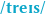substances

status measured difficulty not learned 37% [default] 0

#### Parent (intermediate) annotation

Open it
Other components of blood include plasma (around 55 percent) and other trace substances, including white blood cells and platelets.

#### Original toplevel document (pdf)

cannot see any pdfs

#### Flashcard 4773088988428

Tags
#blood #has-images #medicine
Question
Other components of blood include plasma (around 55 percent) and other trace substances, including white blood cells and [płytki].
platelets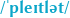status measured difficulty not learned 37% [default] 0

#### Parent (intermediate) annotation

Open it
Other components of blood include plasma (around 55 percent) and other trace substances, including white blood cells and platelets.

#### Original toplevel document (pdf)

cannot see any pdfs

#### Annotation 4773092920588

#blood #medicine
Plasma is a pale-yellow liquid which keeps everything else in suspension, and moving freely within your body. Just like an efficient drainage system, plasma carries waste, like toxic chemicals, nutrients
and hormones, away from cells. After a night out pub-hopping, you can thank your plasma for helping you out.

#### pdf

cannot see any pdfs

#### Flashcard 4773096066316

Tags
#blood #medicine
Question
Plasma is [ blado-żółty płyn] which keeps everything else in suspension, and moving freely within your body. Just like an efficient drainage system, plasma carries waste, like toxic chemicals, nutrients
and hormones, away from cells. After a night out pub-hopping, you can thank your plasma for helping you out.
a pale-yellow liquid

status measured difficulty not learned 37% [default] 0

#### Parent (intermediate) annotation

Open it
Plasma is a pale-yellow liquid which keeps everything else in suspension, and moving freely within your body. Just like an efficient drainage system, plasma carries waste, like toxic chemicals, nutrients and hormones

#### Original toplevel document (pdf)

cannot see any pdfs

#### Flashcard 4773099736332

Tags
#blood #medicine
Question
Plasma is a pale-yellow liquid which keeps everything else [ w zawieszeniu], and moving freely within your body. Just like an efficient drainage system, plasma carries waste, like toxic chemicals, nutrients
and hormones, away from cells. After a night out pub-hopping, you can thank your plasma for helping you out.
in suspension

status measured difficulty not learned 37% [default] 0

#### Parent (intermediate) annotation

Open it
Plasma is a pale-yellow liquid which keeps everything else in suspension, and moving freely within your body. Just like an efficient drainage system, plasma carries waste, like toxic chemicals, nutrients and hormones, away from cells. After a night out pub-h

#### Original toplevel document (pdf)

cannot see any pdfs

#### Flashcard 4773102619916

Tags
#blood #has-images #medicine
Question
Plasma is a pale-yellow liquid which keeps everything else in suspension, and moving freely within your body. Just like an efficient [system kanalizacyjny], plasma carries waste, like toxic chemicals, nutrients and hormones, away from cells. After a night out pub-hopping, you can thank your plasma for helping you out.
drainage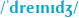system

status measured difficulty not learned 37% [default] 0

#### Parent (intermediate) annotation

Open it
Plasma is a pale-yellow liquid which keeps everything else in suspension, and moving freely within your body. Just like an efficient drainage system, plasma carries waste, like toxic chemicals, nutrients and hormones, away from cells. After a night out pub-hopping, you can thank your plasma for helping you out.

#### Original toplevel document (pdf)

cannot see any pdfs

#### Flashcard 4773108124940

Tags
#blood #medicine
Question
Plasma is a pale-yellow liquid which keeps everything else in suspension, and moving freely within your body. Just like an efficient drainage system, plasma carries waste, like toxic chemicals, nutrients
and hormones, away from cells. After a night out [włóczenie się po pubach],you can thank your plasma for helping you out.
pub-hopping

status measured difficulty not learned 37% [default] 0

#### Parent (intermediate) annotation

Open it
in suspension, and moving freely within your body. Just like an efficient drainage system, plasma carries waste, like toxic chemicals, nutrients and hormones, away from cells. After a night out <span>pub-hopping, you can thank your plasma for helping you out. <span>

#### Original toplevel document (pdf)

cannot see any pdfs

#### Annotation 4773112057100

#blood #medicine
White blood cells are an important part of the body’s immune system. Like knights in shining armour, they attack viruses and other nasties to keep you healthy and on your feet.

#### pdf

cannot see any pdfs

#### Flashcard 4773114416396

Tags
#blood #has-images #medicine
Question
White blood cells are an important part of the body’s immune system. [jak rycerz w ślniącej zbroi], they attack viruses and other nasties to keep you healthy and on your feet.
Like knights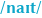in shining armour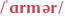status measured difficulty not learned 37% [default] 0

#### Parent (intermediate) annotation

Open it
White blood cells are an important part of the body’s immune system. Like knights in shining armour, they attack viruses and other nasties to keep you healthy and on your feet.

#### Original toplevel document (pdf)

cannot see any pdfs

#### Annotation 4773121494284

#blood #medicine
Platelets are what help your skin heal after a cut or a scrape. Once they are out on the surface they form a clever web of protein which stops further blood flow and allows the skin to mend itself.

#### pdf

cannot see any pdfs

#### Flashcard 4773123591436

Tags
#blood #has-images #medicine
Question
Platelets are what help your skin [goić] after a cut or a scrape. Once they are out on the surface they form a clever web of protein which stops further blood flow and allows the skin to mend itself.
heal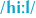status measured difficulty not learned 37% [default] 0

#### Parent (intermediate) annotation

Open it
Platelets are what help your skin heal after a cut or a scrape. Once they are out on the surface they form a clever web of protein which stops further blood flow and allows the skin to mend itself.

#### Original toplevel document (pdf)

cannot see any pdfs

#### Flashcard 4773130669324

Tags
#blood #has-images #medicine
Question
Platelets are what help your skin heal after a cut or a scrape. Once they are out on the surface they form a clever web of protein which stops further blood flow and allows the skin to [naprawiać, zdrowieć] itself.
mend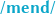status measured difficulty not learned 37% [default] 0

#### Parent (intermediate) annotation

Open it
n> Platelets are what help your skin heal after a cut or a scrape. Once they are out on the surface they form a clever web of protein which stops further blood flow and allows the skin to mend itself. <span>

#### Original toplevel document (pdf)

cannot see any pdfs

#### Annotation 4773137485068

#blood #medicine
Interestingly, blood is made deep within your body. The bone marrow inside your bones produces blood at an impressive rate. Red blood cells are completely changed every 120 days on average. This means that every single blood cell of the five litres in your body is less than four months old.

#### pdf

cannot see any pdfs

#### Flashcard 4773140368652

Tags
#blood #has-images #medicine
Question
Interestingly, blood is made deep within your body. The [szpik kosny] inside your bones produces blood at an impressive rate. Red blood cells are completely changed every 120 days on average. This means that every single blood cell of the five litres in your body is less than four months old.
bone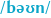marrowstatus measured difficulty not learned 37% [default] 0

#### Parent (intermediate) annotation

Open it
Interestingly, blood is made deep within your body. The bone marrow inside your bones produces blood at an impressive rate. Red blood cells are completely changed every 120 days on average. This means that every single blood cell of the five litres in y

#### Original toplevel document (pdf)

cannot see any pdfs

#### Flashcard 4773146135820

Tags
#blood #has-images #medicine
Question
Interestingly, blood is made deep within your body. The bone marrow inside your bones produces blood at an impressive rate. Red blood cells are completely changed every 120 days [średnio, przeciętnie]. This means that every single blood cell of the five litres in your body is less than four months old.
on average